## Applications of Second‐Order Equations

Skydiving. The principal quantities used to describe the motion of an object are position ( s), velocity ( v), and acceleration ( a). Since velocity is the time derivative of the position, and acceleration is the time derivative of the velocity, acceleration is the second time derivative of the position. Therefore, the position function st) for a moving object can be determined by writing Newton's Second Law, F net = ma, in the form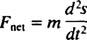and solving this second‐order differential equation for s.

[You may see the derivative with respect to time represented by a dot. For example, ⋅ (“ s dot”) denotes the first derivative of s with respect to t, and(“ s double dot”) denotes the second derivative of s with respect tot. The dot notation is used only for derivatives with respect to time.]

Example 1: A sky diver (mass m) falls long enough without a parachute (so the drag force has strength kv 2) to reach her first terminal velocity (denoted v 1). When her parachute opens,the air resistance force has strengthKv. At what minimum altitude must her parachute open so that she slows to within 1% of her new (much lower) terminal velocity ( v 2) by the time she hits the ground?

Let y denote the vertical distance measured downward form the point at which her parachute opens (which will be designated time t = 0). Then Newton's Second Law ( F net = ma) becomes mg – Kv = ma, or, since v =and a =,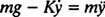This situation is therefore described by the IVPThe differential equation is second‐order linear with constant coefficients, and its corresponding homogeneous equation is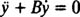where B = K/m. The auxiliary polynomial equation, r 2 = Br = 0, has r = 0 and r = −B as roots. Since these are real and distinct, the general solution of the corresponding homogeneous equation isThe given nonhomogeneous equation has y = ( mg/Kt as a particular solution, so its general solution isNow, to apply the initial conditions and evaluate the parameters c 1 and c 2:These two equations implyOnce these values are substituted into (*), the complete solution to the IVP can be written asThe derivative of this expression gives the velocity of the sky diver t seconds after the parachute opens: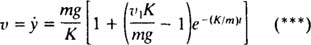The question asks for the minimum altitude at which the sky diver's parachute must be open in order to land at a velocity of (1.01) v 2. Therefore, set v equal to (1.01) v 2 in equation (***) and solve for t; then substitute the result into (**) to find the desired altitude. Omitting the messy details, once the expression in (***) is set equal to (1.01) v 2, the value of t is found to be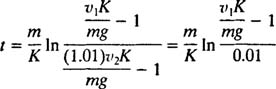and substituting this result into (**) yieldsTo evaluate the numerical answer, the following values are used:

mass of sky diver: m = 70 kg

gravitational acceleration: g = 9.8 m/s 2

air resistance proportionality constant:  K = 110 kg/s

terminal velocity without parachute:terminal velocity with parachute:These substitutions give a descent time t [the time interval between the parachute opening to the point where a speed of (1.01) v 2 is attained] of approximately 4.2 seconds, and a minimum altitude at which the parachute must be opened of y ≈ 55 meters (a little higher than 180 feet).

Simple harmonic motion. Consider a spring fastened to a wall, with a block attached to its free end at rest on an essentially frictionless horizontal table. The block can be set into motion by pulling or pushing it from its original position and then letting go, or by striking it (that is, by giving the block a nonzero initial velocity). The force exerted by the spring keeps the block oscillating on the tabletop. This is the prototypical example ofsimple harmonic motion.

The force exerted by a spring is given by Hooke's Law; this states that if a spring is stretched or compressed a distance x from its natural length, then it exerts a force given by the equation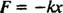The positive constant k is known as the spring constant and is directly realted to the spring's stiffness: The stiffer the spring, the larger the value of k. The minus sign implies that when the spring is stretched (so that x is positive), the spring pulls back (because F is negative), and conversely, when the spring is compressed (so that x is negative), the spring pushes outward (because F is positive). Therefore, the spring is said to exert arestoring force, since it always tries to restore the block to its equilibrium position (the position where the spring is neither stretched nor compressed). The restoring force here is proportional to the displacement ( F = −kx α x), and it is for this reason that the resulting periodic (regularly repeating) motion is called simple harmonic.

Newton's Second Law can be applied to this spring‐block system. Once the block is set into motion, the only horizontal force that acts on it is the restoring force of the spring. Therefore, the equation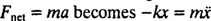or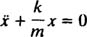This is a homogeneous second‐order linear equation with constant coefficients. The auxiliary polynomial equation is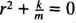, which has distinct conjugate complex rootsTherefore, the general solution of this differential equation is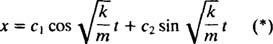This expression gives the displacement of the block from its equilibrium position (which is designated x = 0).

Example 2: A block of mass 1 kg is attached to a spring with force constant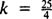N/m. It is pulled 310 m from its equilibrium position and released from rest. Obtain an equation for its position at any time t; then determine how long it takes the block to complete one cycle (one round trip).

All that is required is to adapt equation (*) to the present situation. First, since the block is released from rest, its intial velocity is 0:Since c 2 = 0, equation (*) reduces toNow, since x(0) = + 310m, the remaining parameter can be evaluated: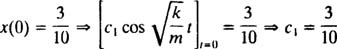Finally, sinceandTherefore, the equation for the position of the block as a function of time is given by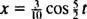where x is measured in meters from the equilibrium position of the block. This function is periodic, which means it repeats itself at regular intervals. The cosine and sine functions each have a period of 2π, which means every time the argument increases by 2π, the function returns to its previous value. (Recall that if, say, x = cosθ, then θ is called the argument of the cosine function.) The argument here is 52 t, and 52 t will increase by 2π every time t increases by 45π. Therefore, this block will complete one cycle, that is, return to its original position ( x = 310 m), every 4/5π ≈ 2.5 seconds.

The length of time required to complete one cycle (one round trip) is called the period of the motion (and denoted by T.) It can be shown in general that for the spring‐block oscillator,Note that the period does not depend on where the block started, only on its mass and the stiffness of the spring. The maximum distance (greatest displacement) from equilibrium is called the amplitude of the motion. Therefore, it makes no difference whether the block oscillates with an amplitude of 2 cm or 10 cm; the period will be the same in either case. This is one of the defining characteristics of simple harmonic motion: the period is independent of the amplitude.

Another important characteristic of an oscillator is the number of cycles that can be completed per unit time; this is called the frequency of the motion [denoted traditionally by v (the Greek letter nu) but less confusingly by the letter f]. Since the period specifies the length of time per cycle, the number of cycles per unit time (the frequency) is simply the reciprocal of the period: f = 1/ T. Therefore, for the spring‐block simple harmonic oscillator,Frequency is usually expressed in hertz (abbreviated Hz); 1 Hz equals 1 cycle per second.

The quantity √ km (the coefficient of t in the argument of the sine and cosine in the general solution of the differential equation describing simple harmonic motion) appears so often in problems of this type that it is given its own name and symbol. It is called the angular frequency of the motion and denoted by ω (the Greek letter omega). Note that ω = 2π f.

Damped oscillations. The spring‐block oscillator is an idealized example of a frictionless system. In real life, however, frictional (or dissipative) forces must be taken into account, particularly if you want to model the behavior of the system over a long period of time. Unless the block slides back and forth on a frictionless table in a room evacuated of air, there will be resistance to the block's motion due to the air (just as there is for a falling sky diver). This resistance would be rather small, however, so you may want to picture the spring‐block apparatus submerged in a large container of clear oil. The viscosity of the oil will have a profound effect upon the block's oscillations. The air (or oil) provides a damping force, which is proportional to the velocity of the object. (Again, recall the sky diver falling with a parachute. At the relatively low speeds attained with an open parachute, the force due to air resistance was given as Kv, which is proportional to the velocity.)

With a restoring force given by − kx and a damping force given by − Kv (the minus sign means that the damping force opposes the velocity), Newton's Second Law ( F net = ma) becomes − kx − Kv = ma, or, since v =and a =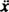This second‐order linear differential equation with constant coefficients can be expressed in the more standard form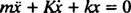The auxiliary polynomial equation is mr 2 + Kr + k = 0, whose roots areThe system will exhibit periodic motion only if these roots are distinct conjugate complex numbers, because only then will the general solution of the differential equation involve the periodic functions sine and cosine. In order for this to be the case, the discriminant K 2 – 4 mk must be negative; that is, the damping constant K must be small; specifically, it must be less than 2 √ mk . When this happens, the motion is said to beunderdamped, because the damping is not so great as to prevent the system from oscillating; it just causes the amplitude of the oscillations to gradually die out. [If the damping constant K is too great, then the discriminant is nonnegative, the roots of the auxiliary polynomial equation are real (and negative), and the general solution of the differential equation involves only decaying exponentials. This implies there would be no sustained oscillations.]

In the underdamped case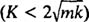, the roots of the auxiliary polynomial equation can be written asand consequently, the general solution of the defining differential equation isExample 3: (Compare to Example 2.) A block of mass 1 kg is attached to a spring with force constantN/m. It is pulled 310m from its equilibrium position and released from rest. If this spring‐block apparatus is submerged in a viscous fluid medium which exerts a damping force of – 4 v (where v is the instantaneous velocity of the block), sketch the curve that describes the position of the block as a function of time.

The net force on the block is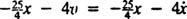, so Newton's Second Law becomesbecause m = 1. Since the roots of the auxiliary polynomial equation,, are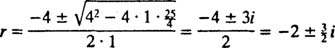the general solution of the differential equation isBecause the block is released from rest, v(0) =(0) = 0:This implies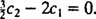And, since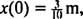,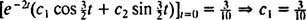Therefore,and the equation that gives the position of the block as a function of time iswhere x is measured in meters from the equilibrium position of the block.

This expression for the position function can be rewritten using the trigonometric identity cos(α – β) = cos α cos β + sin α sin β, as follows: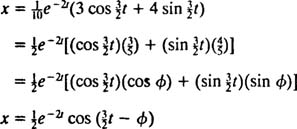The phase angle, φ, is defined here by the equations cos φ = 35 and sin φ = 45, or, more briefly, as the first‐quadrant angle whose tangent is 43 (it's the larger acute angle in a 3–4–5 right triangle). The presence of the decaying exponential factor e −2 t in the equation for xt) means that as time passes (that is, as t increases), the amplitude of the oscillations gradually dies out. See Figure .Figure 1

The angular frequency of this periodic motion is the coefficient of t in the cosine,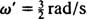, which implies a period ofCompare this to Example 2, which described the same spring, block, and initial conditions but with no damping. The position function there was x = 310 cos 52 t; it had constant amplitude, an angular frequency of ω = 5/2 rad/s, and a period of just 45 π ≈ 2.5 seconds. Therefore, not only does (under) damping cause the amplitude to gradually die out, but it also increases the period of the motion. But this seems reasonable: Damping reduces the speed of the block, so it takes longer to complete a round trip (hence the increase in the period). This will always happen in the case of underdamping, since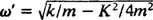will always be lower than.

Electric circuits and resonance. When an electric circuit containing an ac voltage source, an inductor, a capacitor, and a resistor in series is analyzed mathematically, the equation that results is a second‐order linear differentically equation with constant coefficients. The voltage vt) produced by the ac source will be expressed by the equation v = V sin ω t, where V is the maximum voltage generated. An inductor is a circuit element that opposes changes in current, causing a voltage drop of Ldidt), where i is the instantaneous current and L is a proportionality constant known as the inductance. A capacitor stores charge, and when each plate carries a magnitude of charge q, the voltage drop across the capacitor is q/C, where C is a constant called the capacitance. Finally, a resistor opposes the flow of current, creating a voltage drop equal to iR, where the constant R is the resistance. Kirchhoff's Loop Rule states that the algebraic sum of the voltage differences as one goes around any closed loop in a circuit is equal to zero. Therefore, if the voltage source, inductor, capacitor, and resistor are all in series, thenwhich can be rewritten as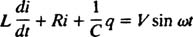Now, if an expression for it)—the current in the circuit as a function of time—is desired, then the equation to be solved must be written in terms of i. To this end, differentiate the previous equation directly, and use the definition i = dqdt:This differential equation governs the behavior of an LRC series circuit with a source of sinusoidally varying voltage.

The first step in solving this equation is to obtain the general solution of the corresponding homogeneous equation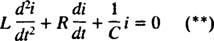But notice that this differential equation has exactly the same mathematical form as the equation for the damped oscillator,By comparing the two equations, it is easy to see that the current ( i) is analogous to the position (x), the inductance ( L) is analogous to the mass ( m), the resistance ( R) is analogous to the damping constant ( K), and the reciprocal capacitance (1/ C) is analogous to the spring constant ( k). Since the general solution of (***) was found to be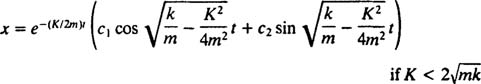the general solution of (**) must be, by analogy,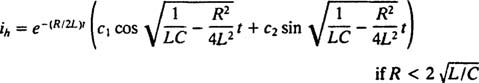But the solution does not end here. The original differential equation (*) for the LRC circuit was nonhomogeneous, so a particular solution must still be obtained. The family of the nonhomogeneous right‐hand term, ω V cos ω t, is {sin ω t, cos ω t}, so a particular solution will have the form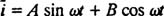where A and B are the undeteremined coefficinets. Given this expression for i , it is easy to calculate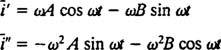Substituting these last three expressions into the given nonhomogeneous differential equation (*) yields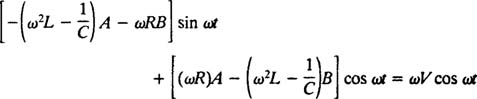Therefore, in order for this to be an identity, A and B must satisfy the simultaneous equations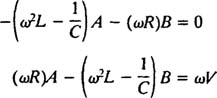The solution of this system is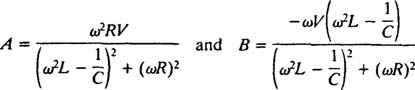These expressions can be simplified by invoking the following standard definitions:

• ω L is called the inductive reactance and denoted X L
•is called the capacitive reactance and denoted X c
• X L – X c is simply called the reactance and denoted X
•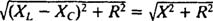is called the impedance and denoted Z

Therefore,and the expressions for the preceding coefficients A and B can be written as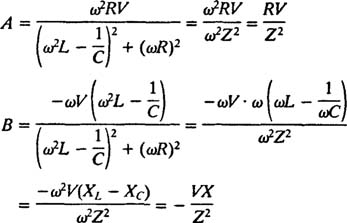These simplifications yield the following particular solution of the given nonhomogeneous differential equation:Combining this with the general solution of the corresponding homogeneous equation gives the complete solution of the nonhomo‐geneous equation: i = h i or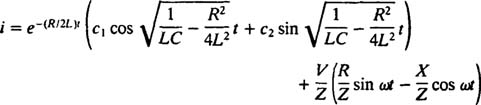Despite its rather formidable appearance, it lends itself easily to analysis. The first term [the one with the exponential‐decay factor e −( R/2 Lt ] goes to zero as t increases, while the second term remains indefinitely. For these reasons, the first term is known as the transient current, and the second is called the steady‐state current:Example 4: Consider the previously covered underdamped LRC series circuit. Once the transient current becomes so small that it may be neglected, under what conditions will the amplitude of the oscillating steady‐state current be maximized? In particular, assuming that the inductance L, capacitance C, resistance R, and voltage amplitude V are fixed, how should the angular frequency ω of the voltage source be adjusted to maximized the steady‐state current in the circuit?

The steady‐state curent is given by the equation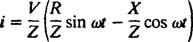By analogy with the phase‐angle calculation in Example 3, this equation is rewritten as follows: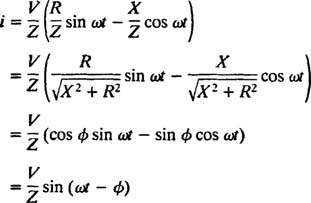(where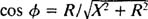andTherefore, the amplitude of the steady‐state current is VZ, and, since V is fixed, the way to maximize VZ is to minimize Z. Because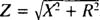Z will be minimized if X = 0. And because ω is necessarily positive,This value of ω is called the resonant angular frequency. When the underdamped circuit is “tuned” to this value, the steady‐state current is maximized, and the circuit is said to be in resonance. This is the principle behind tuning a radio, the process of obtaining the strongest response to a particular transmission. In this case, the frequency (and therefore angular frequency) of the transmission is fixed (an FM station may be broadcasting at a frequency of, say, 95.5 MHz, which actually means that it's broadcasting in a narrow band around 95.5 MHz), and the value of the capacitance C or inductance L can be varied by turning a dial or pushing a button. According to the preceding calculation, resonance is achieved when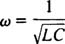Therefore, in terms of a (relatively) fixed ω and a variable capacitance, resonance will occur when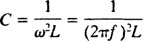(where f is the frequency of the broadcast). Or in terms of a variable inductance, the circuitry will resonate to a particular station when L is adjusted to the value